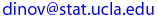## PIC 20 A,    Summer 2002

JAVA and Internet Programming, Computation & Visualization

# Homework Project  3

Instructions: You need to have at least 4 problems completed to get a 100% for this assignment. You are encouraged, however, to finish all (7) problems by the end of the quarter.
• [Project 3_1]  State whether each of the following is true or false. If false, explain why [Proj3_1.txt].
• To start a Java application you need an HTML file that instructs the Java VM how to initialize the program.
• When using an import of the form javax.swing.*, all classes in the package are imported.
• You do not need import statements if the full package name and class name are specified each time you refer to a class in a program.
• If the class C is a sub-class of B, which is itself a subclass of another class A, then C inherits functionality from B, but not from the superclass A.

• [Project 3_2]  Write an applet that inputs three floating-point numbers from the user and displays the sum, average, product, the smallest and the largest of these numbers as strings on the applet [Proj3_2.java, Proj3_2.class , Proj3_2.html].

• [Project 3_3]  Write an applet that inputs from the user the radius of a circle as a floating-point number and draws the circle’s diameter, circumference and area, as strings. You may use the predefined constant Math.PI for the value of pi. This constant is more precise than the value 3.14159. Class Math is defined in the java.lang package, so you do not need to import it. Use the following formulas (r is the radius):  diameter = 2r, circumference = 2 pi r, area = pi r2. [Proj3_3.java, Proj3_3.class , Proj3_3.html].

• [Project 3_4]  Write an applet that draws a box, an oval, an arrow and a diamond using asterisks (*) as follows [Proj3_4.java, Proj3_4.class , Proj3_4.html].
 *********          ***              *              * *       *        *     *           ***            * * *       *       *       *         *****          *   * *       *       *       *           *           *     * *       *       *       *           *          *       * *       *       *       *           *           *     * *       *       *       *           *            *   * *       *        *     *            *             * * *********          ***              *              *

• [Project 3_5]  Write an applet that reads integers until "0" is entered and determines and prints the largest and smallest integers in the group and the group average. [Proj3_5.java, Proj3_5.class , Proj3_5.html].

• [Project 3_6]  Write an applet that allows the user to input the four arguments required by method drawOval, then draws an oval using the four input values. Think about how would you go about having the oval move in the window? How about bounce back inward as it hits one of the four sides of the drawing panel? [Proj3_6.java, Proj3_6.class , Proj3_6.html].

• [Project 3_7]  Write an applet that calculates the squares and cubes of the numbers from 0 to 10 and draws the resulting values in table format as follows:

 number square cube 0 0 0 1 1 1 2 4 8 3 9 27 4 16 64 5 25 125 6 36 216 7 49 343 8 64 512 9 81 729 10 100 1000

[Note: This program does not require any input from the user. Proj3_7.java, Proj3_7.class , Proj3_7.html].

 Assignment 3, due date:   Wed., July 17, 2002 Last modified on by.

\Ivo D. Dinov, Ph.D., Departments of Statistics, Neurology, Program in Computing, UCLA School of Medicine/